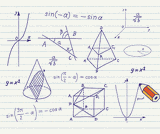# 4 Results

View
Selected filters:
• OH.TMM.0002.Core.4Conditional Remix & Share Permitted
CC BY-NC
Rating

The Pre-Calculus course was developed through the Ohio Department of Higher Education OER Innovation Grant. This work was completed and the course was posted in September 2019. The course is part of the Ohio Transfer Module and is also named TMM002. For more information about credit transfer between Ohio colleges and universities, please visit: www.ohiohighered.org/transfer.Team LeadKameswarrao Casukhela                   Ohio State University LimaContent ContributorsLuiz Felipe Martins                             Cleveland State UniversityIeda Rodrigues                                   Cleveland State UniversityTeri Thomas                                        Stark State CollegeLibrarianDaniel Dotson                                     Ohio State University                     Review TeamAlice Taylor                                        University of Rio GrandeRita Ralph                                          Columbus State Community College

Subject:
Applied Science
Education
Mathematics
Calculus
Physical Science
Material Type:
Full Course
Provider:
Ohio Open Ed Collaborative
01/09/2019Conditional Remix & Share Permitted
CC BY-NC
Rating

Trigonometric Equations, trigonometric identitiesTMM 002 PRECALCULUS (Revised March 21, 2017)4c. Become fluent with conversions using traditional equivalency families.*(e.g., (sin(𝑡))2+(cos(𝑡))2=1; (tan(𝑡))2+1=(sec(𝑡))2; sums/differences; products; double angle; Euler’s Formula (𝑒𝑖𝜃=cos(𝜃)+𝑖sin(𝜃)); etc.)Sample Tasks:The student can prove trigonometric identities.The student solves trigonometric equations.To solve √cos(4𝑡) = √sin(4𝑡), the student solves cos(4𝑡) =sin(4𝑡) and knows this procedure may result in extraneous solutions.The student solves |cos (2𝜃−3)| + 32 = 2 by rewriting the left-hand side as a piecewise-defined function.The student can rewrite formulas involving multiple occurrences of the variable to formulas involving a single occurrence. Write 𝑎sin(𝑤 𝑡)+𝑏cos(𝑤 𝑡) as 𝐴 sin (𝑤 𝑡+𝐵) or 𝐵 cos (𝑤 𝑡+𝐵). The student can rewrite sums as products to reveal attributes such as zeros, envelopes, and phase interference.The student can solve 2 𝑠𝑖𝑛2(𝑡)+7sin(𝑡)−4=0 on a given interval.The student can solve 𝑙𝑜𝑔4(sin (𝑡))+𝑙𝑜𝑔4(2sin(𝑡)+7)=1 on a given interval.

Subject:
Mathematics
Calculus
Trigonometry
Material Type:
Module
Provider:
Ohio Open Ed Collaborative
05/28/2019Conditional Remix & Share Permitted
CC BY-NC
Rating

Complex numbers, Euler's notation, sine-cosine representation, DeMoivre's Theorem, product and quotient of complext numbers, powers and roots of complex numbers, magnitude, polar coordinates,

Subject:
Mathematics
Calculus
Trigonometry
Material Type:
Module
Provider:
Ohio Open Ed Collaborative
05/28/2019Unrestricted Use
CC BY
Rating

Instructor lecture notes for a college level PreCalculus Course. Videos are embedded.

Subject:
Mathematics
Material Type:
Instructional Guide
Provider:
Ohio Dominican University
Author:
Anna Davis
Anna Davis